Chapter 1 Class 10 Real Numbers

Class 10
Important Questions for Exam - Class 10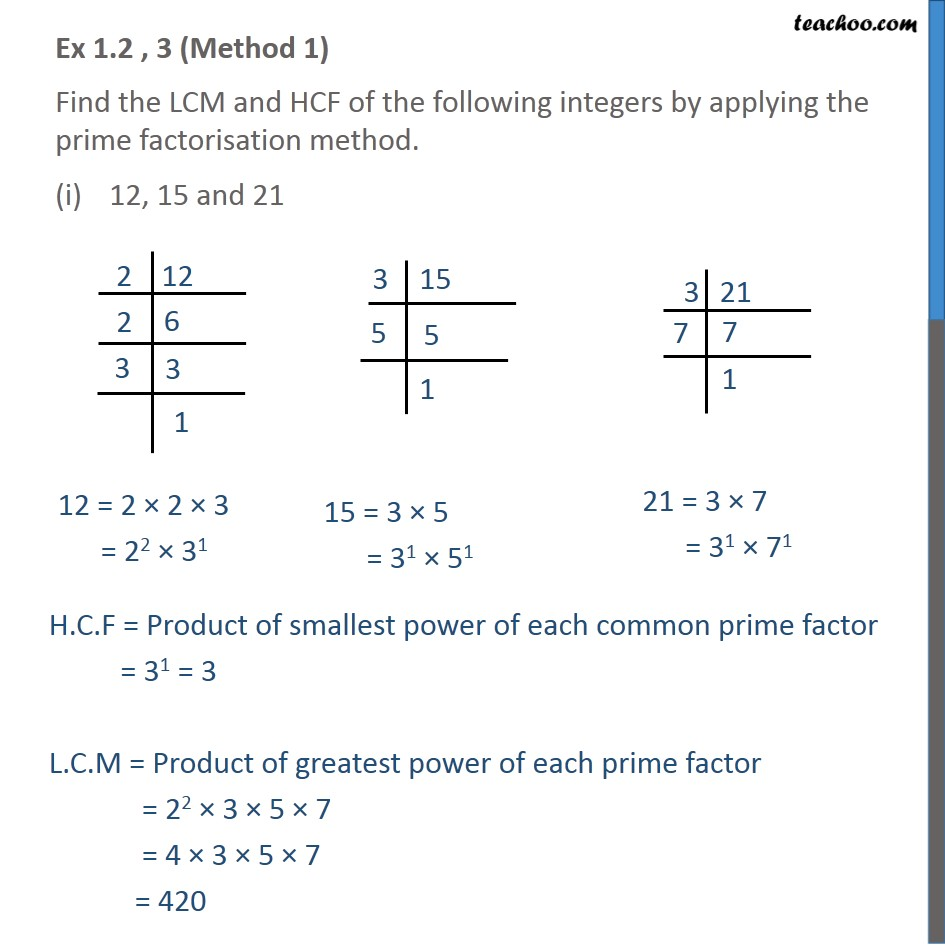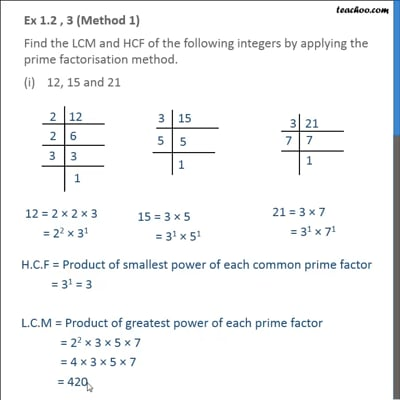This video is only available for Teachoo black users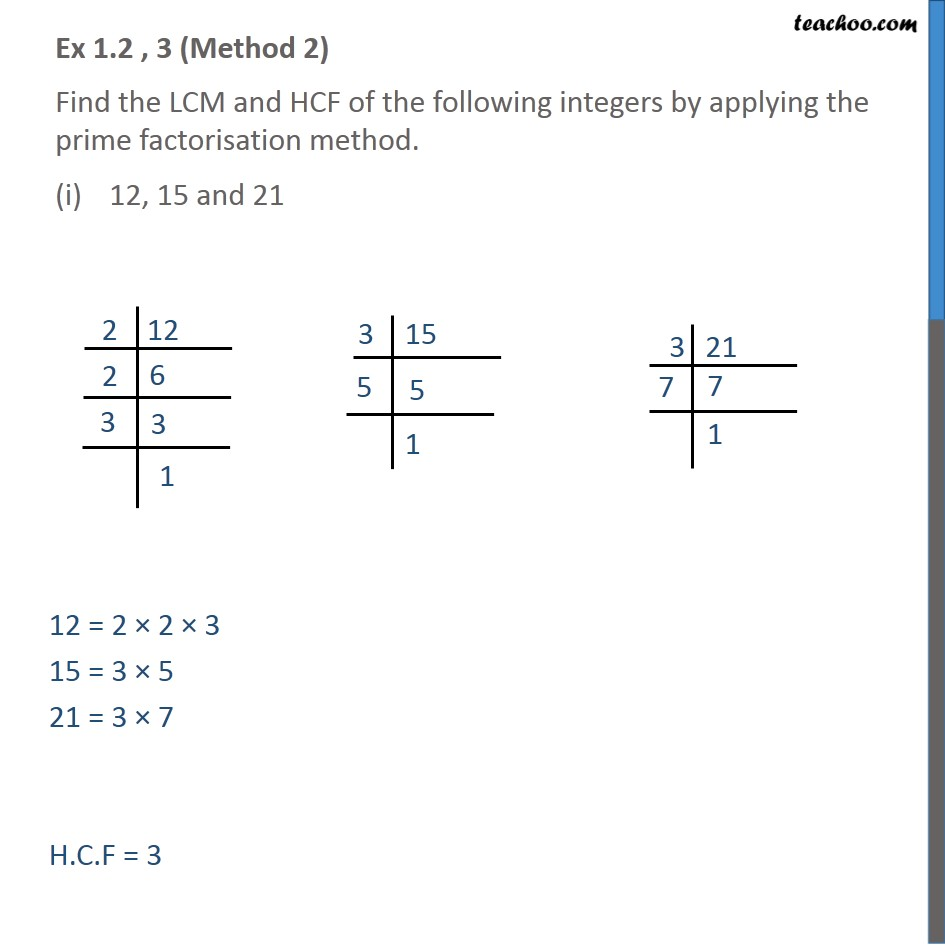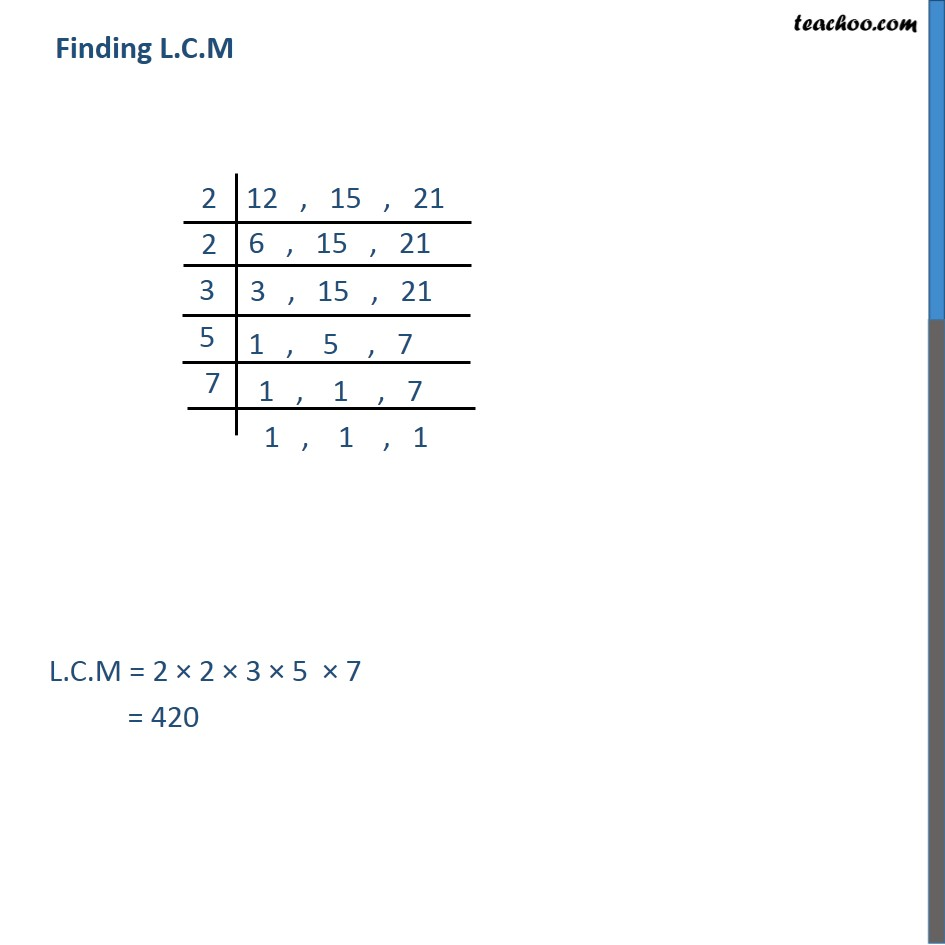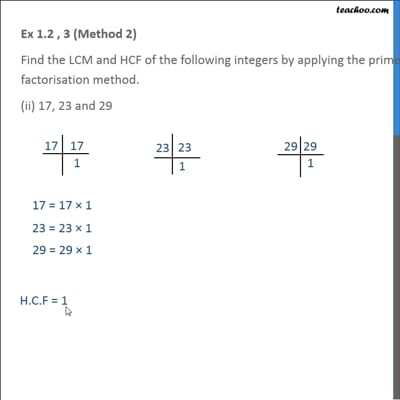This video is only available for Teachoo black users

Introducing your new favourite teacher - Teachoo Black, at only ₹83 per month

### Transcript

Ex 1.2 , 3 (Method 1) Find the LCM and HCF of the following integers by applying the prime factorisation method. 12, 15 and 21 H.C.F = Product of smallest power of each common prime factor = 31 = 3 L.C.M = Product of greatest power of each prime factor = 22 × 3 × 5 × 7 = 4 × 3 × 5 × 7 = 420 Ex 1.2 , 3 (Method 2) Find the LCM and HCF of the following integers by applying the prime factorisation method. 12, 15 and 21 12 = 2 × 2 × 3 15 = 3 × 5 21 = 3 × 7 H.C.F = 3 Finding L.C.M L.C.M = 2 × 2 × 3 × 5 × 7 = 420